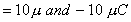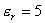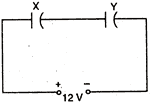Wednesday 12th August 2020

CBSE Guess > Papers > Question Papers > Class XII > 2004 > Physics > Delhi Set-III

PHYSICS (Set III—Delhi)

Except for the following questions all the remaining question have been asked in Set I and Set II.

Q. 5. ‘Microwaves are used in Radar.’ Why? 1

Q. 9. Two point chargesare separated by a distance of 40 cm in air. (i) Calculate the electrostatic potential energy of the system, assuming the zero of the potential energy to be at infinity. (ii) Draw an equipotential surface of the system. 2

Q. 10. An astronomical telescope, in normal adjustment position has magnifying power 5. The distance between the objective and the eye-piece is 120 cm. Calculate the focal lengths of the objective and of the eye-piece. 2

Or

A compound microscope with an objective of 2.0 cm focal length and an eye-piece of 4.0 cm focal length, his a tube length of 40 cm. Calculate the magnifying power of the microscope, if the final image is formed at the near point of the eye.

Q. 11. With the help of an example, explain, how the neutron to proton ratio changes during alpha decay of a nucleus. 2

Q. 12. Find the wavelength of electromagnetic waves of frequency 6 X 1012Hz in free space. Give its two applications. 2

Q. 14. X and Y are two parallel plate capacitors having the same area of plates and same separation between the plates. ’X has are between the plates and Y contains a dielectric medium of.(i) Calculate the potential difference between the plates of X and Y.
(ii) What lithe ratio of electrostatic energy stored In X and Y’ 3

Q. 16. Draw the energy band diagrams of p-type and n-type semiconductors.
A semiconductor has equal electron and hole concentration 6 X 108 m-3
On doping with a certain impurity, electron concentration increases to 8 X 1012 m-3.
Identify the type of semiconductor after doping. 3

 Physics 2004 Question Papers Class XII Delhi Outside Delhi Compartment Delhi Compartment Outside DelhiSet ISet ISet ISet ISet IISet IISet IISet IISet IIISet III

CBSE 2004 Question Papers Class XII